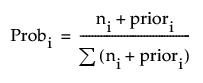Predictive and Specialized Modeling > Partition Models > Statistical Details for the Partition Platform > Predicted Probabilities in Decision Tree and Bootstrap Forest
Publication date: 11/10/2021

## Predicted Probabilities in Decision Tree and Bootstrap Forest

The predicted probabilities for the Decision Tree and Bootstrap Forest methods are calculated as described below by the Prob statistic.

For categorical responses in Decision Tree, the Show Split Prob command shows the following statistics:

Rate

The proportion of observations at the node for each response level.

Prob

The predicted probability for that node of the tree. The method for calculating Prob for the ith response level at a given node is defined as follows:where the summation is across all response levels; ni is the number of observations at the node for the ith response level; and priori is the prior probability for the ith response level, calculated as follows:

priori = λpi+ (1-λ)Pi

where pi is the priori from the parent node, Pi is the Probi from the parent node, and λ is a weighting factor currently set at 0.9.

The method for calculating Prob assures that the predicted probabilities are always nonzero.